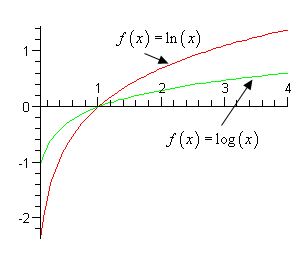Paul's Online Notes
Home / Algebra Trig Review / Exponentials & Logarithms / Logarithm Properties
Show Mobile Notice Show All Notes Hide All Notes
Mobile Notice
You appear to be on a device with a "narrow" screen width (i.e. you are probably on a mobile phone). Due to the nature of the mathematics on this site it is best views in landscape mode. If your device is not in landscape mode many of the equations will run off the side of your device (should be able to scroll to see them) and some of the menu items will be cut off due to the narrow screen width.

### Section 3.3 : Logarithm Properties

Complete the following formulas. Show All Solutions Hide All Solutions

1. $${\log _b}b =$$
Show Solution
${\log _b}b = 1\hspace{0.5in}{\rm{because}}\hspace{0.5in}{b^1} = b$
2. $${\log _b}1 =$$
Show Solution
${\log _b}1 = 0\hspace{0.5in}{\rm{because}}\hspace{0.5in}{b^0} = 1$
3. $${\log _b}{b^x} =$$
Show Solution
${\log _b}{b^x} = x$
4. $${b^{{{\log }_b}x}} =$$
Show Solution
${b^{{{\log }_b}x}} = x$
5. $${\log _b}xy =$$
Show Solution
${\log _b}xy = {\log _b}x + {\log _b}y$

THERE IS NO SUCH PROPERTY FOR SUMS OR DIFFERENCES!!!!!

\begin{align*}{\log _b}\left( {x + y} \right) & \ne {\log _b}x + {\log _b}y\\ {\log _b}\left( {x - y} \right) & \ne {\log _b}x - {\log _b}y\end{align*}
6. $${\log _b}\left( {\frac{x}{y}} \right) =$$
Show Solution
${\log _b}\left( {\frac{x}{y}} \right) = {\log _b}x - {\log _b}y$

THERE IS NO SUCH PROPERTY FOR SUMS OR DIFFERENCES!!!!!

\begin{align*}{\log _b}\left( {x + y} \right) &\ne {\log _b}x + {\log _b}y\\ {\log _b}\left( {x - y} \right) &\ne {\log _b}x - {\log _b}y\end{align*}
7. $${\log _b}\left( {{x^r}} \right) =$$
Show Solution
${\log _b}\left( {{x^r}} \right) = r{\log _b}x$

Note in this case the exponent needs to be on the WHOLE argument of the logarithm. For instance,

${\log _b}{\left( {x + y} \right)^2} = 2{\log _b}\left( {x + y} \right)$

However,

${\log _b}\left( {{x^2} + {y^2}} \right) \ne 2{\log _b}\left( {x + y} \right)$
8. Write down the change of base formula for logarithms.
Show Solution

The change of base formula for logarithms is,

${\log _b}x = \frac{{{{\log }_a}x}}{{{{\log }_a}b}}$

This is the most general change of base formula and will convert from base $$b$$ to base $$a$$. However, the usual reason for using the change of base formula is so you can compute the value of a logarithm that is in a base that you can’t easily compute. Using the change of base formula means that you can write the logarithm in terms of a logarithm that you can compute. The two most common change of base formulas are

${\log _b}x = \frac{{\ln x}}{{\ln b}}\hspace{0.5in}{\rm{and}}\hspace{0.5in}{\rm{lo}}{{\rm{g}}_{\rm{b}}}x = \frac{{\log x}}{{\log b}}$

In fact, often you will see one or the other listed as THE change of base formula!

In the problems in the Basic Logarithm Functions section you computed the value of a few logarithms, but you could do these without the change of base formula because all the arguments could be written in terms of the base to a power. For instance,

${\log _7}49 = 2\hspace{0.5in}{\rm{because}}\hspace{0.5in}{7^2} = 49$

However, this only works because 49 can be written as a power of 7! We would need the change of base formula to compute $${\log _7}50$$.

${\log _7}50 = \frac{{\ln 50}}{{\ln 7}} = \frac{{3.91202300543}}{{1.94591014906}} = 2.0103821378$

OR

${\log _7}50 = \frac{{\log 50}}{{\log 7}} = \frac{{1.69897000434}}{{0.845098040014}} = 2.0103821378$

So, it doesn’t matter which we use, you will get the same answer regardless.

Note as well that we could use the change of base formula on $${\log _7}49$$ if we wanted to as well.

${\log _7}49 = \frac{{\ln 49}}{{\ln 7}} = \frac{{3.89182029811}}{{1.94591014906}} = 2$

This is a lot of work however, and is probably not the best way to deal with this.

9. What is the domain of a logarithm?
Show Solution

The domain $$y = {\log _b}x$$ is $$x > 0$$. In other words, you can’t plug in zero or a negative number into a logarithm. This makes sense if you remember that $$b > 0$$ and write the logarithm in exponential form.

$y = {\log _b}x\hspace{0.25in} \Rightarrow \hspace{0.25in}{b^y} = x$

Since $$b > 0$$ there is no way for $$x$$ to be either zero or negative. Therefore, you can’t plug a negative number or zero into a logarithm!

10. Sketch the graph of $$f\left( x \right) = \ln \left( x \right)$$ and $$g\left( x \right) = \log \left( x \right)$$.
Show Solution

Not much to this other than to use a calculator to evaluate these at a few points and then make the sketch. Here is the sketch.From this graph we can see the following behaviors of each graph.

\begin{align*} & \ln \left( x \right) \to \infty {\mbox{ as }}x \to \infty & \hspace{0.5in} & {\rm{and}} & \hspace{0.5in} & \ln \left( x \right) \to - \infty {\mbox{ as }}x \to 0\,\,\left( {x > 0} \right)\\ & \log \left( x \right) \to \infty {\mbox{ as }}x \to \infty & \hspace{0.5in} & {\rm{and}} & \hspace{0.5in} & \log \left( x \right) \to - \infty {\mbox{ as }}x \to 0\,\,\left( {x > 0} \right)\end{align*}

Remember that we require $$x > 0$$ in each logarithm.